Courses

# Lakhmir Singh & Manjit Kaur: Sources of Energy, Solutions- 4 Class 10 Notes | EduRev

## Class 10 : Lakhmir Singh & Manjit Kaur: Sources of Energy, Solutions- 4 Class 10 Notes | EduRev

The document Lakhmir Singh & Manjit Kaur: Sources of Energy, Solutions- 4 Class 10 Notes | EduRev is a part of the Class 10 Course Lakhmir Singh & Manjit Kaur Solutions: Class 10 Science.
All you need of Class 10 at this link: Class 10

Page No:156

Question 1: What type of nuclear reaction is responsible for the liberation of energy (a) in a nuclear reactor ?
(b) in the sun ?

Solution : (a) Nuclear fission
(b) Nuclear fusion

Question 2: Which product of the nuclear fission of uranium-235 is utilised to cause further fission of its nuclei ?

Solution : Neutrons.

Question 3: Which particles bring about the fission of uranium-235 ?

Solution : Neutrons.

Question 4: State whether the fission of uranium-235 is caused by low energy neutrons or high energy neutrons.

Solution : By the low energy neutrons.

Question 5: Name the type of nuclear reaction which is involved in the working of:
(a) a hydrogen bomb.
(b) an atom bomb.

Solution : (a) Nuclear fusion.
(b) Nuclear fission.

Question 6: Name the moderator used in a nuclear reactor.

Solution : Graphite.

Question 7: Of what material are the control rods of a nuclear reactor made ?

Solution : Boron.

Question 8: What do you think is the purpose of the thick, concrete chamber surrounding the reactor of a nuclear power plant ?

Solution : Thick concrete chamber is required to prevent the radioactive rays to travel outside.

Page No:157

Question 9: Where, in a nuclear power station, is uranium, used up ?

Solution : Uranium is used up in the reactor.

Question 10: State one use of nuclear fission reactions.

Solution : Nuclear fission reactions are used for generating electricity at a nuclear power plant.

Question 11: Name the unit which is commonly used for expressing the energy released in nuclear reactions.

Solution : Million electron volt (MeV)

Question 12: How many MeV are equivalent to 1 atomic mass unit (u) ?

Solution : 1 atomic mass unit=931 MeV

Question 13: Fill in the following blanks with suitable words :
(a) Splitting of a heavy nucleus into two lighter nuclei is called…………
(b) Uranium-235 atoms will split when hit by……… This is called……………….
(c) Nuclear………. is used in nuclear power stations for the production of electricity.
(d) In a nuclear power station, nuclear fission takes place in the…………

Solution : (a) nuclear fission
(b) neutrons; nuclear fission
(c) fission
(d) reactor

Question 14: What is nuclear fission ? Explain with an example. Write the equation of the nuclear reaction involved.

Solution : The process in which the heavy nucleus of a radioactive atom(such as uranium) splits up into smaller nuclei when bombarded with low energy neutrons, is called nuclear fission.
E.g., When uranium-235 atoms are bombarded with slow moving neutrons, the heavy uranium nucleus breaks up to produce two medium-weighted atoms and 3 neutrons, with the emission of tremendous amount of energy.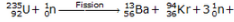tremendous amount of energy

Question 15: (a) What is nuclear fusion ? Explain with an example. Write the equation of the reaction involved.
(b) Why are very high temperatures required for fusion to occur ?

Solution : (a) The process in which two nuclei of light elements (like that of hydrogen) combine to form a heavy nucleus (like that of helium), is called nuclear fusion.
When deuterium atoms are heated to an extremely high temperature under extremely high pressure, then two deuterium nuclei combine together to form a heavy nucleus of helium, and a neutron is emitted. A tremendous amount of energy is liberated in the process.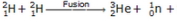tremendous amount of energy

(b) Because very high energy is required to force the lighter nuclei (which repel each other) to fuse together to form a bigger nuclei.

Question 16: What is the nuclear fuel in the sun ? Describe the process by which energy is released in the sun. Write the equation of the nuclear reaction involved.

Solution : The nuclear fuel in the sun is hydrogen gas.
The sun can be considered as a big thermonuclear furnace where hydrogen atoms continuously get fused into helium atoms. Mass gets lost during these fusion reactions and energy is being produced.
Nuclear reaction: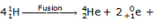tremendous amount of energy

Question 17: (a) Write Einstein’s mass-energy equation. Give the meaning of each symbol which occurs in it.
(b) If 25 atomic mass units (u) of a radioactive material are destroyed in a nuclear reaction, how much energy is released in MeV ?

Solution : (a) Einstein’s equation: E=mc2
where E is the amount of energy produced if mass m is destroyed, and c is the speed of light in vacuum.
(b) 1 atomic mass unit = 931 MeV
25 atomic mass unit = 931 x 25 MeV = 23275 MeV
23275 MeV of energy is released.

Question 18: (a) What is the source of energy of ths sun and other stars ?
(b) Describe the working of a hydrogen bomb.
(c) What is common between the sun and a hydrogen bomb ?

Solution : (a) Nuclear fusion reactions 0f hydrogen.
(b) The hydrogen bomb consists of heavy isotopes of hydrogen (deuterium and tritium) alongwith lithium-6. The explosion of hydrogen bomb is done by using an atom bomb. When the aton bomb is exploded, its fission reaction produces a lot of heat which raises the temperature of deuterium and tritium to 107oC in a few microseconds. Then fusion reactions of deuterium and tritium take place producing a tremendous amount of energy. This explodes the hydrogen bomb. Lithium-6 is used to produce more tritium needed for fusion.
(c) The source of energy is same for both the sun and the hydrogen atom, i.e. nuclear fusion.

Question 19: (a) What will happen if slow moving neutrons are made to strike the atoms of a heavy element 92U235 ? What
is the name of this process ?
(b) Write a nuclear equation to represent the process which takes place.
(c) Name one installation where such a process is utilised.

Solution : (a) When slow moving neutrons are made to strike the atoms of a heavy element uranium-235, the heavy uranium nucleus breaks up to produce two medium-weighted atoms and 3 neutrons, with the emission of tremendous amount of energy. This process is called nuclear fission.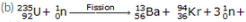tremendous amount of energy

(c) Nuclear Power Station

Question 20: (a) What are the advantages of nuclear energy ?
(b) State the disadvantages of nuclear energy.

Solution : (a) Advantages of nuclear energy:
(i) It produces a large amount of useful energy from a very small amount of a nuclear fuel.
(ii) Once the nuclear fuel is loaded into the reactor, the nuclear power plant can go on producing electricity for two to three years at a stretch. There is no need of feeding the fuel again and again.
(iii) It does not produce gases like CO2 or SO2.
(i) The waste products of nuclear fission reactions are radioactive which keep on emitting harmful radiations for thousands of years and are difficult to store or dispose safely.
(ii) Very high cost of installation is required.
(iii) There is a limited availability of uranium fuel.

Question 21: The following questions are about the nuclear reactor of a power plant.
(a) Which isotope of uranium produces the energy in the fuel rods ?
(b) Will the fuel rods last for ever ?
(c) Is the energy produced by nuclear fission or nuclear fusion ?
(d) What is the purpose of using the graphite moderator ?
(e) What is the function of boron rods in the nuclear reactor ?
(f) Why is liquid sodium (or carbon dioxide gas) pumped through the reactor ?

Solution : (a) Uranium-235
(b) No
(c) Nuclear fission
(d) Moderator slows down the speed of neutrons to make them fit for causing fission.
(e) Boron rods are used to absorb excess neutrons and prevent the fission reaction from going out of control.
(f) Liquid sodium or carbon dioxide gas is used as a ‘coolant’ to transfer the heat produced to heat exchanger for converting water into steam.

Question 22: In the reactor of a nuclear power plant, name the material which is used :
(a) as a moderator
(c) in the fuel rods
(d) in the control rods
(e) to carry away heat

Solution : (a) Graphite
(b) Concrete
(c) Uranium-235
(d) Boron
(e) Liquid sodium

Question 23: In the nuclear reactor of a power plant:
(a) how do control rods control the rate of fission ?
(b) how is heat removed from the reactor core, and what use is made of this heat ?

Solution : (a) Control rods control the rate of fission by absorbing the excess neutrons and preventing the fission reaction from going out of control.
(b) Heat is removed from the nuclear reactor core with the help of liquid sodium, which absorbs the heat and transfers it to the heat exhanger. This heat is used for converting water in the heat exchanger into steam, which is then used to produce electricity by rotating a turbine and its shaft connected to a generator.

Question 24: How does inserting the control rods in the graphite core affect the fission in the reactor ?

Solution : On inserting the control rods in the graphite core, the rods start absorbing the excess neutrons and maintain the rate of reaction as per requirement. The rods can be raised or lowered in the reactor from outside. The part which is inside the reactor absorbs neutrons.

Question 25: What are the advantages and disadvantages of using nuclear fuel for generating electricity ?

Solution : Advantages of using nuclear fuel: Electricity can be produced for almost two to three years with the same uranium fuel in a nuclear power plant.
Disadvantages of using nuclear fuel: The nuclear wastes produced by the fission of uranium-235 during the generation of electricity are radioactive and extremely harmful.

Question 26: (a) What is a nuclear reactor ? What is the fuel used in a nuclear reactor ?
(b) With the help of a labelled diagram, describe the working of a nuclear power plant.
(c) How is the working nuclear reactor of a power plant shut down in an emergency ?
(d) Name five places in India where nuclear power plants are located.

Solution : (a) Nuclear reactor is a device designed to maintain a chain reaction producing a steady flow of neutrons generated by the fission of heavy nuclei. Uranium-235 is used as a fuel in a nuclear reactor.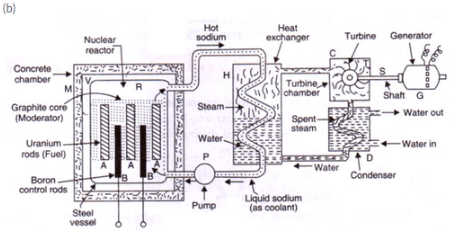In a nuclear power plant, the fission of uranium-235 is carried out in a reactor R. Uranium-235 rods are inserted in a graphite core which acts as a moderator to slow down the neutrons. Boron rods B absorb excess neutrons and controls the rate of reaction. Liquid sodium or carbon dioxide gas, which is pumped continuously through pipes embedded in reactor by using a pump P, is used as a ‘coolant’ to transfer the heat produced to heat exchanger for converting water into steam. The hot steam at high pressure goes into a turbine chamber and makes the turbine rotate. The shaft of the generator also rotates and drives a generator connected to it.
(c) By inserting the boron control rods fully into the reactor.
(d) Five places in India where nuclear powerplants are located are:
(i) Tarapur.
(ii) Kalpakkam.
(iii) Narora.
(iv) Kaprapur.
(vi) kaiga.

Page No:158

Question 27:
(a) Differentiate between nuclear fission and nuclear fusion.
(b) Which of the two, nuclear fission and nuclear fusion, is made use of :
(i) for the production of electricity ?
(ii) for making a hydrogen bomb ?
(c) Which produces more energy : nuclear fusion or nuclear fission ?
(d) Calculate the energy released in joules when 5 g of a material is completely converted into energy during a nuclear reaction.
(e) How much is this energy in MeV ? (Speed of light = 3 × 108 m/s)
Solution :

(a)

 Nuclear Fission Nuclear Fusion Natural occurrence of the process: Fission reaction does not normally occur in nature. Fusion occurs in stars, such as the sun Byproducts of the reaction: Fission produces many highly radioactive particles. Few radioactive particles are produced by fusion reaction, but if a fission ' trigger' is used, radioactive particles will result from that. Energy Ratios: The energy released by fission is a million times greater than that released in chemical reactions: but lower than the energy released by nuclear fusion The energy released by fusion is three to four times greater than the energy released by fission Nuclear weapon: One class of nuclear weapon is a fission bomb, also known as an atomic bomb or atom bomb. One class of nuclear weapon is the hydrogen bomb, which uses a fission reaction to 'trigger' a fusion reaction Energy requirement: Takes little energy to split two atoms in a fission reaction Extremely high energy is required to bring two or more protorts close enough that nuclear forces overcome their electrostatic repulsio.

(b) (i) Nuclear fission
(ii) Nuclear fusion
(c) Nuclear fusion
(d)Mass.m = 5g = 0.005kg Speedof light, c=3x108m/s

We know that, E= mc2
E = 0.005x(3x108)2
E = 4.5x1014.J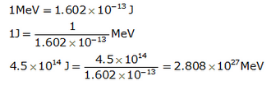Page No:159

Question 46: A nuclear reaction is represented by the following equation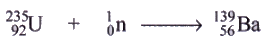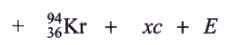(a) Name the process represented by this equation and describe what takes place in this reaction.
(b) Identify the particle c and the number x of such particles produced in the reaction.
(c) What does E represent ?
(d) Name one installation where the above nuclear reaction is utilised.
(e) What type of bomb is based on similar type of reactions ?

Solution : (a) Nuclear fission; a large nucleus splits into two smaller nuclei with the release of energy, brought about by the absorption of a neutron.
(b) Particles c is neutron; x=3
(c) Energy liberated
(d) Nuclear power station
(e) Atom bomb

Question 47: A nuclear reaction is represented by the equation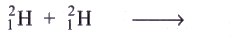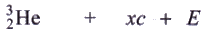(a) Name the process represented by this equation and describe what happens during this reaction.
(b) Identify the particle c and the number x of such particles produced in the reaction.
(c) What does £ represent ?
(d) State two conditions under which such a reaction takes place.
(e) What type of nuclear bomb is based on similar reactions ?

Solution : (a) Nuclear fusion; two smaller nuclei combine to form a bigger nucleus with the release of energy, brought about under the conditions of high temperature and pressure.
(b) Particle c is neutron; x=1
(c) Energy liberated
(d) Millions of degree of temperature and millions of pascals of pressure.
(e) Hydrogen bomb.

Question 48: The mass numbers of four elements A, B, C and D are 2, 20, 135 and 235, respectively. Which one of them will be most suitable to make : (i) an atom bomb, and (it)a hydrogen bomb ?

Solution : (i) D(mass number 235)
(ii) A(mass number2)

Question 49: A nuclear power plant is working normally. What would you do if the reactor core suddenly got too hot ?

Solution : We will insert the control rods of boron a little more into the reactor to reduce the rate of nuclear fission process.

Question 50: A nuclear reactor has half the length of all its control rods inserted in graphite. What must be done so that the reactor produces more heat ?

Solution : The control rods should be withdrawn a little more from inside the reactor. This will increase the rate of nuclear fission process and hence more heat.

Question 51: Explain why, in a nuclear reactor, the chain reaction stops if the control rods are fully inserted into the graphite.

Solution : The control rods absorb all the neutrons, stopping the nuclear chain reaction.

Page No:161

Question 1: Which of the two is a cleaner fuel : hydrogen or CNG ? Why ?

Solution : Hydrogen is a cleaner fuel because burning of hydrogen produces only water. which is harmless; but burning of CNG produces CO2 and water. CO2 produces greenhouse effect.

Question 2: Which of the two is more energy efficient: filament type electric bulb or CFL ? Why ?

Solution : CFL is more energy efficient because it wastes much less energy as heat than filament type electric bulb.

Question 3: How long are the energy resources of the earth like coal, petroleum and natural gas expected to last ?

Solution : Coal – 200 years
Petroleum – 40 years
Natural Gas – 60 years

Question 4: Name two devices which can be utilised for the cooking of food so as to save fuel .

Solution : Solar cooker; pressure cooker

Question 5: What are the various factors which we should keep in mind while choosing a source of energy ?

Solution : Various factors to be kept in mind while choosing a source of energy are:
1. the ease of extracting energy from that source
2. the cost of extracting energy from that source
3. the efficiency of technology available for extracting energy from that source
4. the damage to environment caused by using that source

Question 6: Can any source of energy be pollution free ? Explain your answer with an example.

Solution : No. No source of energy is pollution free.

For e.g., the use of wind generators is pollution-free but the processes involved in making it must have damaged the

Question 7: What are the environmental consequences of the increasing demand for energy ?

Solution : The environmental consequences of the increasing the demand for energy are:
(i) The combustion of fossil fuels is producing acid rain and damaging plants, soil and aquatic life.
(ii) The combustion of fossil fuels is increasing the green house effect.
(iii) Nuclear power plants are increasing radioactivity in the environment.
(iv) Construction of hydro-power plants is disturbing the ecological balance.

Question 8: What steps would you suggest to reduce energy consumption ?

Solution : Steps for reducing the energy consumption:-
(i) Switch off all the lights, fans, TV and other appliances when not needed.
(ii) Use of biogas as cooking fuel should be encouraged.
(iii) Bicycles should be used for short distances to save precious fuels like petrol.
(iv) Solar cookers should be used to cook food.

Offer running on EduRev: Apply code STAYHOME200 to get INR 200 off on our premium plan EduRev Infinity!

61 docs

,

,

,

,

,

,

,

,

,

,

,

,

,

,

,

,

,

,

,

,

,

,

,

,

;